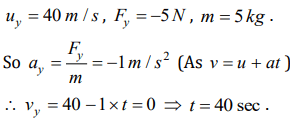## Laws of Motion Questions and Answers Part-10

1. A man of weight 80 kg is standing in an elevator which is moving with an acceleration of $6m\diagup s^{2}$  in upward direction. The apparent weight of the man will be $\left(g=10 m\diagup s^{2}\right)$
a) 1480 N
b) 1280 N
c) 1380 N
d) None of these

Explanation: The apparent weight of man, R = m(g + a) = 80(10 + 6) = 1280 N

2. A force of 100 dynes acts on a mass of 5 gram for 10 sec. The velocity produced is
a) 2000 cm / sec
b) 200 cm / sec
c) 20 cm / sec
d) 2 cm / sec

Explanation: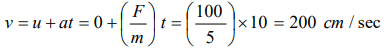3. When the speed of a moving body is doubled
a) Its acceleration is doubled
b) Its momentum is doubled
c) Its kinetic energy is doubled
d) Its potential energy is doubled

Explanation: Its momentum is doubled

4. A body of mass m collides against a wall with a velocity v and rebounds with the same speed. Its change of momentum is
a) 2 mv
b) mv
c) – mv
d) Zero

Explanation: $\triangle$ p = pi - pf = mv-(-mv) = 2mv

5. A thief stole a box full of valuable articles of weight W and while carrying it on his back, he jumped down a wall of height ‘h’ from the ground. Before he reached the ground he experienced a load of
a) 2W
b) W
c) W/2
d) Zero

Explanation: In the condition of free fall apparent weight becomes zero.

6. N bullets each of mass m kg are fired with a velocity v $m s^{-1}$ at the rate of n bullets per second upon a wall. The reaction offered by the wall to the bullets is given by
a) nmv
b) $\frac{Nmv}{n}$
c) $n\frac{Nm}{v}$
d) $n\frac{Nv}{m}$

Explanation: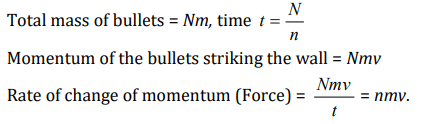7. If a body of mass m is carried by a lift moving with an upward acceleration a, then the forces acting on the body are (i) the reaction R on the floor of the lift upwards (ii) the weight mg of the body acting vertically downwards. The equation of motion will be given by
a) $R=mg- ma$
b) $R=mg+ ma$
c) $R=ma- mg$
d) $R=mg\times ma$

Explanation: $R = mg + ma$

8. With what minimum acceleration can a fireman slides down a rope while breaking strength of the rope is$\frac{2}{3}$ of his weight
a) $\frac{2}{3}g$
b) g
c) $\frac{1}{3}g$
d) Zero

Explanation: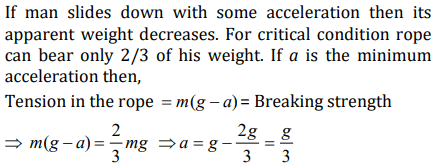9. The velocity of a body at time t = 0 is $10\sqrt{2}m\diagup s$   in the north-east direction and it is moving with an acceleration of $2 m\diagup s^{2}$  directed towards the south. The magnitude and direction of the velocity of the body after 5 sec will be
a) 10 m/s, towards east
b) 10 m/s, towards north
c) 10 m/s, towards south
d) 10 m/s, towards north-east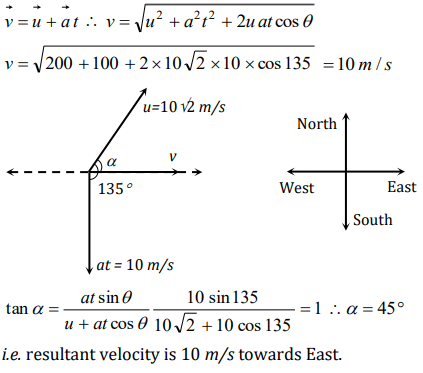10. A body of mass 5 kg starts from the origin with an initial velocity $\vec{u}=30\hat{i}+40\hat{j}ms^{-1}$   . If a constant force $\vec{F}=-\left(\hat{i}+5\hat{j}\right)N$    acts on the body, the time in which the y–component of the velocity becomes zero is